# Constant-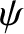Linear Resonant Response Regimes

Suppose that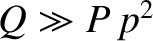and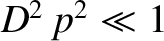. It follows that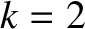,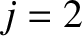,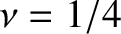, and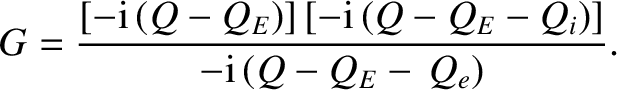(5.92)

Hence, we deduce that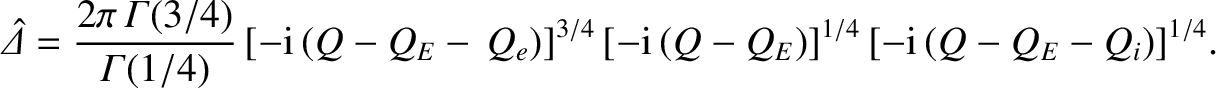(5.93)

This response regime is known as the resistive-inertial regime, because the layer response is dominated by plasma resistivity and ion inertia [2,13,17]. The characteristic layer width is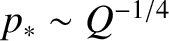, which implies that the regime is valid when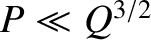,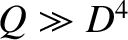,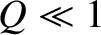, and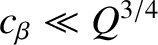.

Suppose that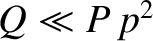and. It follows that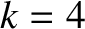,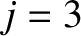,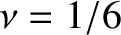, and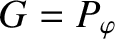. Hence, we deduce that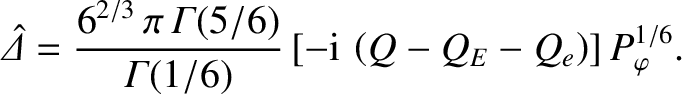(5.94)

This response regime is known as the viscous-resistive regime, because the layer response is dominated by ion perpendicular viscosity and plasma resistivity [3,11]. The characteristic layer width is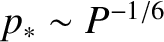, which implies that the regime is valid when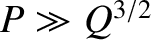,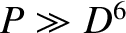,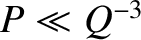, and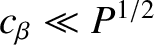.

Suppose thatand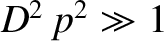. It follows that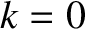,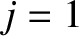,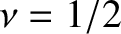, and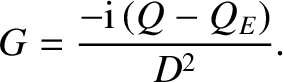(5.95)

Hence, we deduce that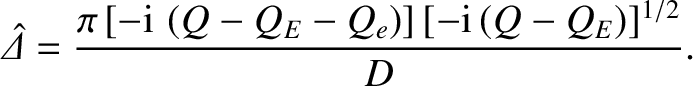(5.96)

This response regime is known as the semi-collisional regime [9,21]. The characteristic layer width is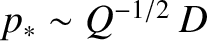, which implies that the regime is valid when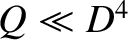,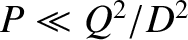,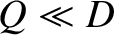, and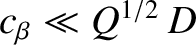.

Suppose, finally, thatand. It follows that,,, and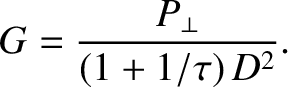(5.97)

Hence, we deduce that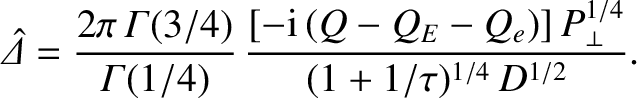(5.98)

This response regime is known as the diffusive-resistive regime, because the layer response is dominated by perpendicular energy diffusivity and plasma resistivity . The characteristic layer width is, which implies that the regime is valid when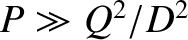,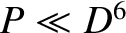,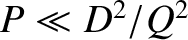, and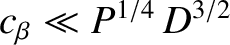.